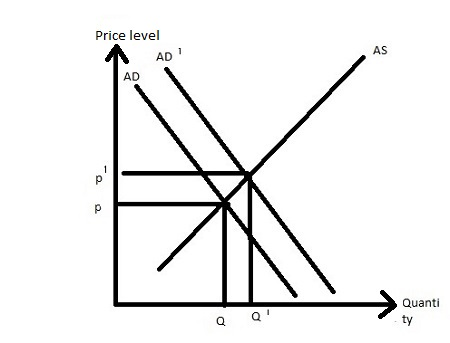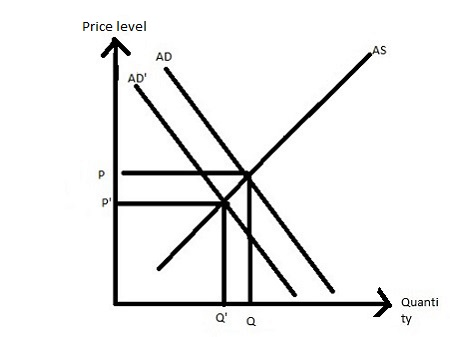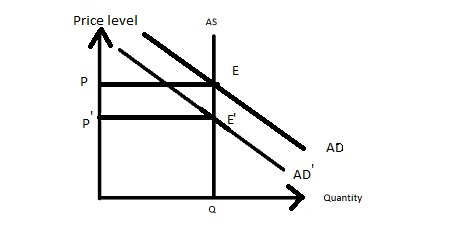# Consider the short run. An investment tax credit is applied to encourage business spending. The...

## Question:

Consider the short run. An investment tax credit is applied to encourage business spending. The result will be in the price level and in output.

a. an increase; a decrease no change;

b. an increase none of the listed options

c. an increase; no change

d. an increase; an increase

In the short run, policy is enacted via a tax increase. The effect on the AD-AS graph would be in prices and _ in output.

a. a decrease; no change

b. an increase; a decrease

c. a decrease; a decrease

d. a decrease; an increase

e. none of the listed options

In the short run, the Fed (Federal Reserve) buys \$100,000 in US government bonds from a bank for policy purposes. When we graph this on the AD-AS framework we see it results in in prices and _ in output.

a. an increase; a decrease

b. a decrease; a decrease

c. none of the listed options

d. an increase; an increase

e. a decrease; an increase

Tight (contractionary) fiscal policy will, in the long run, cause _ in the price level and in output.

a. none of the listed options

b. a decrease; a decrease

c. a decrease; no change

d. a decrease; an increase

e. an increase; a decrease

Consider again the scenario from the previous question. The overall long-run effect of this scenario would be

a. inflation and a decrease in the natural rate of output

b. deflation and no change in the natural rate of output

c. inflation and no change in the natural rate of output

d. deflation and a decrease in the natural rate of output

e. none of the listed options

## Aggregate Demand And Aggregate Supply:

The aggregate demand curve shows the inverse relationship between the price level and the total quantity of all goods demanded. As the price level rises, then the total quantity of all goods demanded falls, and as the price level falls, then the total quantity of all goods demanded rises. This shows that the aggregate demand curve is downward sloping.

The aggregate supply curve shows the direct relationship between the price level and the total quantity of all good supplied. As the price level rises then the total quantity of all goods supplied also rises and as the price level falls then the total quantity of all goods supplied also falls. This shows that the aggregate supply curve is upward sloping.

## Answer and Explanation:

1) An investment tax credit is applied to encourage business spending results in an increase in the aggregate demand with the same aggregate supply curve such that both price level and output increases.Therefore, option (D) an increase; an increase is correct.

2) As the tax increases then the aggregate demand curve shifts leftward with the same aggregate supply curve such that both price level and output decreases.Therefore, Option (C) a decrease; a decrease is correct.

3) If the Fed buys government bonds then the money supply increases in such a way that the rate of interest decreases that increases the investment in such a manner that the aggregate demand curve shifts rightward that increase both price level and output.Therefore, Option (D) an increase; an increase is correct.

4) In the long run, the aggregate supply curve is vertical such that as the government reduces its expenditure or increase taxes then aggregate demand curve shifts leftward with the same aggregate supply curve such that the price level decreases and output remain the same.Therefore, Option (C) a decrease; no change is correct.

5) In the long run, the aggregate supply curve is vertical such that as the government reduces its expenditure or increase taxes then the aggregate demand curve shifts leftward which reduces the price level or deflation and natural rate of output remains the same.

Therefore, Option (B) deflation and no change in the natural rate of output is correct.

#### Learn more about this topic:Aggregate Supply and Aggregate Demand (AS-AD) Model

from Economics 102: Macroeconomics

Chapter 7 / Lesson 3
58K